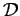## 1. 1. 3 Continuous Problems

A possible interface for the specification of the model obtained is given by continuous physics. Even though there are other approaches possible, most engineering problems can be transformed into continuous problems. In continuous problems a domainis defined and some physical quantities such as velocity or temperature are given as field on the domain. Even though unknown, the solution quantity is assumed to be a field over the continuous domain.

In order to obtain the solution from given quantities some governing relation is given. This can be a simple point-wise operation, a differential equation, or an integral operation such as a convolution.

Continuous problems as they appear in modeling form an interface between modeling and mathematical treatment. In many cases these continuous formulations are used as an interface between two scientists or an interface between a human and a computer, e.g. for the case when mathematical software is used. In each case clearly defined and easily understandable formalisms have to be provided in order to avoid unneccessary overhead and insufficient information at the interface.

Another aspect of interfaces is that information which is passed through (e.g. between an engineer and a mathematician) inevitably changes its semantics. While modelers have a clear idea of the physical behavior of the investigated system, this usually does not hold to a mathematician and is perhaps not even relevant. Moreover, it is necessary to find a discretization scheme which works for the posed equation and yields correct solutions.

Michael 2008-01-16